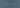# RGB to HSV to RGB - for Shaders

November 15th, 2015 - Software(1 min)TL;DR: Go here to get the RGB->HSV and HSV->RGB conversion functions for shaders.

The other day I tried to tint a colour in a texture (or rather all texture) with a different colour. It’s quite easy to rotate things around, but, if you want to do it parametrically, you’ll need something a bit more complex.

## HSV

The easiest way (?!?!) I could come up with was to shift the hue off all colors using a RGB<->HSV conversion. The algorithm in a shader would be quite simple:

``````for pixel in texture:
rgb = pixel.rgb
hsv = rgb_to_hsv(rgb)
hsv.h += parameter  # parameter is 0-1
if hsv.h > 1:
hsv.h -= 1
pixel.set_color(hsv_to_rgb(hsv))``````

Simple, right?

Now, the two functions are:

1. RGB -> HSV:

``````vec3 rgb2hsv(vec3 c)
{
vec4 K = vec4(0.0, -1.0 / 3.0, 2.0 / 3.0, -1.0);
vec4 p = mix(vec4(c.bg, K.wz), vec4(c.gb, K.xy), step(c.b, c.g));
vec4 q = mix(vec4(p.xyw, c.r), vec4(c.r, p.yzx), step(p.x, c.r));

float d = q.x - min(q.w, q.y);
float e = 1.0e-10;
return vec3(abs(q.z + (q.w - q.y) / (6.0 * d + e)), d / (q.x + e), q.x);
}``````
2. HSV -> RGB:

``````vec3 hsv2rgb(vec3 c)
{
vec4 K = vec4(1.0, 2.0 / 3.0, 1.0 / 3.0, 3.0);
vec3 p = abs(fract(c.xxx + K.xyz) * 6.0 - K.www);
return c.z * mix(K.xxx, clamp(p - K.xxx, 0.0, 1.0), c.y);
}``````

Now, all you have to do is place them in a fragment shader and apply the above algorithm.

The vertex shader is quite basic (done in the OS X’s OpenGL Shader Builder):

``````//1
attribute vec4 a_position;
attribute vec2 a_texCoord;

void main()
{
gl_FrontColor = gl_Color;
gl_TexCoord = gl_MultiTexCoord0;
gl_Position = ftransform();
}``````

The fragment shader is like this (sand the two above methods for brevity)

``````uniform sampler2D tex;
uniform vec3 hue;

// Add the two methods here

void main() {
vec4 textureColor = texture2D(tex, vTextureCoord);
vec3 fragRGB = textureColor.rgb;
vec3 fragHSV = rgb2hsv(fragRGB).xyz;
fragHSV.x += hue.x;
fragHSV.yz *= hue.yz;
fragHSV.xyz = mod(fragHSV.xyz, 1.0);
fragRGB = hsv2rgb(fragHSV);
gl_FragColor = vec4(fragRGB, textureColor.w);
}``````

Yes, it’s like the one from SO, but with the difference that values for hue are between 0 and 1 (rather than 0-360).

Now, all we have to do is to tint only some of the colours… :)

Tagged with
All Tags
Share on

A little experiment: